• 上面的plot格式中,选项是指为了区分多条画出曲线的颜色,线型及标记点而设定的曲线的属性.MATLAB在多组变量绘图时,可将曲线以不同的颜色,不同的线型及标记点表示出来.这些选项如下表所示: (1) 各种颜色属性
Date: 2017/6/6
Author：Soaringlee

多组变量绘图:plot(x1, y1, 选项1, x2, y2, 选项2, ……)
上面的plot格式中,选项是指为了区分多条画出曲线的颜色,线型及标记点而设定的曲线的属性.MATLAB在多组变量绘图时,可将曲线以不同的颜色,不同的线型及标记点表示出来.这些选项如下表所示:
(1) 各种颜色属性选项 'r' 红色      'm' 粉红 'g' 绿色     'c' 青色 'b' 兰色     'w' 白色
'y' 黄色      'k' 黑色

(2) 各种线型属性选项 '-' 实线     '--' 虚线

':' 点线     '-.' 点划线
(3) 各种标记点属性选项 '.' 用点号绘制各数据点        '^' 用上三角绘制各数据点 '+' 用'+'号绘制各数据点      'v' 用下三角绘制各数据点 '*' 用'*'号绘制各数据点       '>' 用右三角绘制各数据点 ' .' 用'.'号绘制各数据点        '<' 用左三角绘制各数据点 's'或square    用正方形绘制各数据点      'p' 用五角星绘制各数据点 'd'或diamond用菱形
展开全文• 这是一个工具箱，可以更轻松地可视化三个变量的向量和标量函数。 大多数基本函数都被重载了。 例如绘图功能。 需要注意的是，使用此工具箱还不能解决任何 PDE。 在这种情况下，您应该使用我的 FEM 工具箱。 有计划...
• xy 散点图，其绘图符号大小与 z 的大小成正比。 在使用不同颜色与后续数据集重叠绘制时，比例因子可实现符号大小的连续性。
• ## matlab的三维绘图和四维绘图

万次阅读 多人点赞 2018-11-12 13:52:55
一、绘图 1.曲线图 plot3(X1,Y1,Z1,...)：以默认线性属性绘制维点集（X1,Y1,Z1）确定的曲线 plot3(X1,Y1,Z1,LineSpec)：以参数LineSpec确定的线性属性绘制维点集 plot3(X1,Y1,Z1,'PropertyName',...
一、三维绘图
1.曲线图
plot3(X1,Y1,Z1,...)：以默认线性属性绘制三维点集（X1,Y1,Z1）确定的曲线plot3(X1,Y1,Z1,LineSpec)：以参数LineSpec确定的线性属性绘制三维点集plot3(X1,Y1,Z1,'PropertyName',PropertyValue,...)：根据指定的属性绘制三维曲线
theta = 0:0.01*pi:2*pi;
x = sin(theta);
y = cos(theta);
z = cos(4*theta);
plot3(x,y,z,'LineWidth',2);
hold on;
theta = 0:0.02*pi:2*pi;
x = sin(theta);
y = cos(theta);
z = cos(4*theta);
plot3(x,y,z,'rd','MarkerSize',10,'LineWidth',2);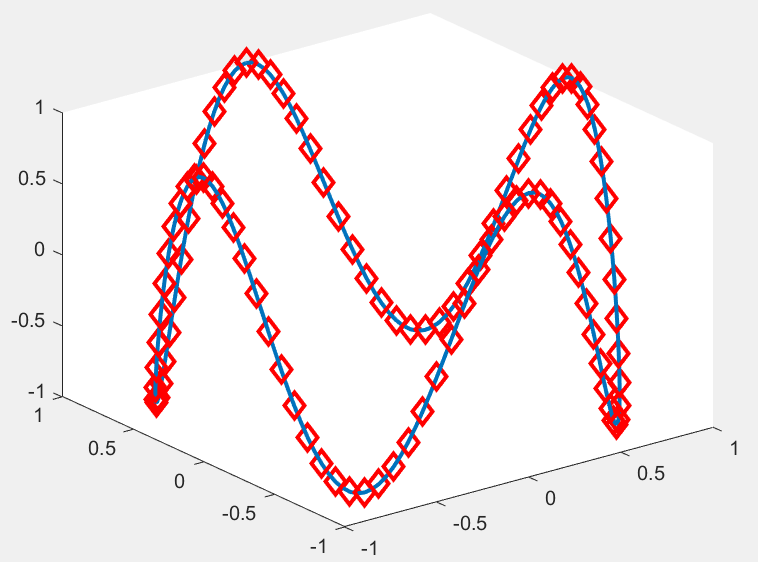2.网格图
绘制函数z=f(x,y)的三维网格图的过程：
确定自变量x和y的取值范围和取值间隔利用meshgrid函数生成“格点”矩阵计算自变量采样“格点”上的函数值：Z = f(x,y)
matlab中提供了mesh函数用于实现绘制网格图：
mesh(X,Y,Z)：绘制三维网格图，颜色与曲面的高度相匹配mesh(Z)：系统默认颜色与网格区域的情况下绘制数据Z的网格图mesh(...,C,'PropertyName',PropertyValue)：对指定的颜色C，指定的属性值，画出三维图形meshc(...)：用于画网格图与基本的等值线图meshz(...)：用于绘制包含零平面的网格图h = mesh(...)：返回图形对象句柄属性值向量h
[X,Y] = meshgrid(-3:.5:3);
Z = 2 * X.^2-3 * Y.^2;
subplot(2,2,1)
plot3(X,Y,Z)
title('plot3')
subplot(2,2,2)
mesh(X,Y,Z)
title('mesh')
subplot(2,2,3)
meshc(X,Y,Z)
title('meshc')
subplot(2,2,4)
meshz(X,Y,Z)
title('meshz')
set(gcf,'Color','w');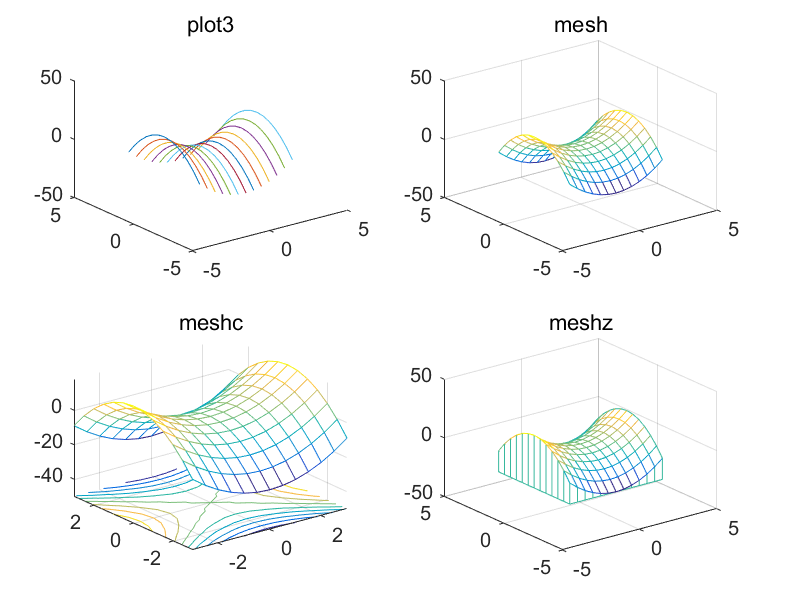3.曲面图
表示三维空间内数据的变化规律。函数有surf、surfc和surfl
surf(X,Y,Z)：绘制三维的彩色曲面图。surf(X,Y,Z,C)：图形的颜色采用参数C,同样可以添加属性值
[x,y] = meshgrid(-3:1/8:3);
z = peaks(x,y);
subplot(2,2,1);surf(z);
title('surf(z)绘制形式');
subplot(2,2,2);surf(x,y,z);
title('surf(x,y,z)绘图形式');
subplot(2,2,3);surfl(x,y,z);
title('surfl(x,y,z)绘图形式');
subplot(2,2,4);surfc(x,y,z);
title('surfc(x,y,z)绘图形式');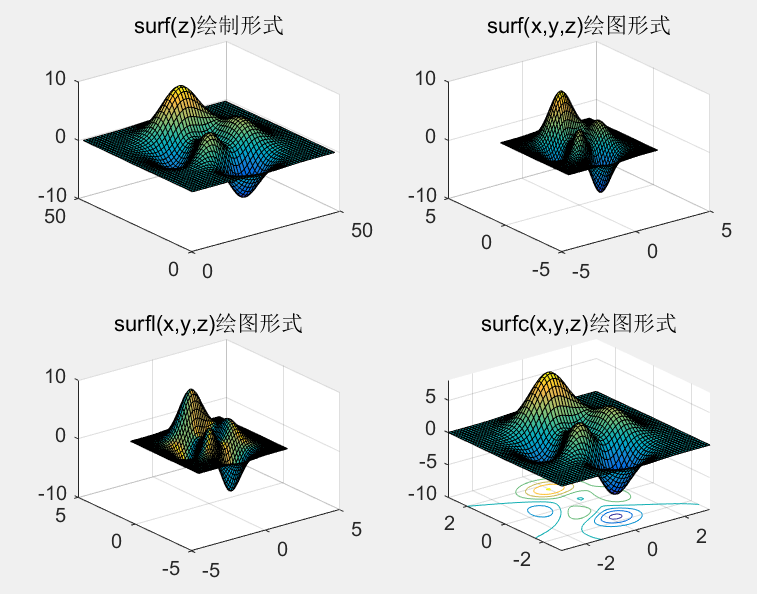4.光照模型
光照是利用方向官员照亮物体的技术，这项技术能使表面微妙的差异更容易看到，光照也能用来对三维的图像增加现实感。
camlight函数：
camlight('light')：在照相机的右上方设置一个光源camlight('light')：在照相机的左上方设置一个光源camlight(az,el)：建立一个相对于照相方位角az与仰角el的光源camlight(...,'style')：设置光源的类型为'local'或‘infinite’light_handle = camlight(...)：返回光源的句柄值
surf(peaks)
axis vis3d
h = camlight('left');
for i = 1:20;
camorbit(10,0)
camlight(h,'left')
pause(.1)
end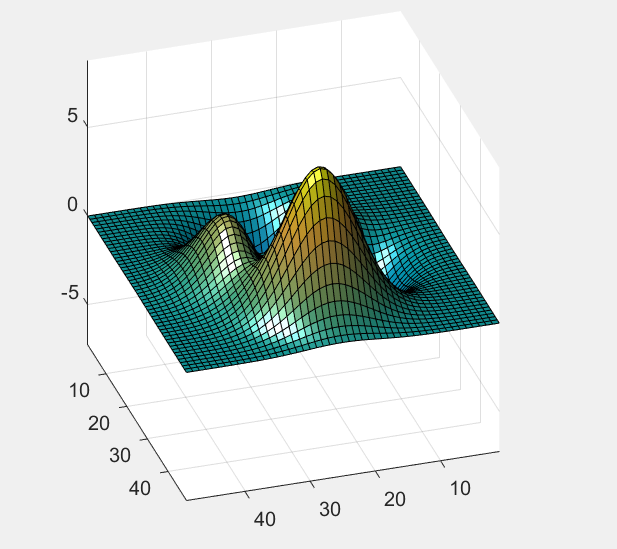light函数：
light('PropertyName',propertyvalue,...)：可设置的光源的属性有color，style，positionhandle = light(...)：返回光源的句柄值
% 准备数据
[X,Y]  = meshgrid(-1:0.1:1);
Z = sin(X.^2.*pi) + cos(Y.*pi);
% 设置无限远平行光源光照效果
subplot(1,2,1);
surf(X,Y,Z);
light('Style','infinit','Position',[0 -0.6 1]);
title('无限远平行光')
% 设置本地光源辐射源光照效果
subplot(1,2,2);
surf(X,Y,Z);
light('Style','local','Position',[0 -0.6 1]);
title('本地辐射光')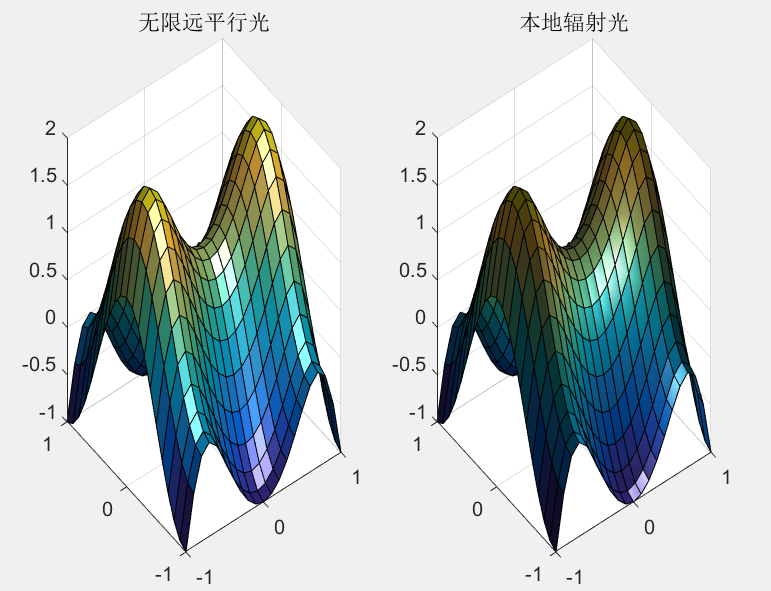lighting函数：
lighting flat：为入射光均匀洒落在图形对象的每个面上，主要与faced配合使用lighting gouraud：先对定点颜色插补，在对定点勾画的面色进行插补，用于曲面表现lighting phong：对定点出的法线插值，在计算个像素的反光，效果好，但费时lighting none：关闭所有光源
[x,y,z] = sphere(25);
subplot(2,2,1);surf(x,y,z);
hold on;
title('lighting none')
subplot(2,2,2);surf(x,y,z);
axis equal;
light('position',[0,0.5 1]);
hold on;
title('lighting flat');
subplot(2,2,3);surf(x,y,z);
axis equal;
light('position',[0,0.5 1]);
hold on;
title('lighting gouraud');
subplot(2,2,4);surf(x,y,z);
axis equal;
light('position',[0,0.5 1]);
hold on;
title('lighting phong');
set(gcf,'color','w');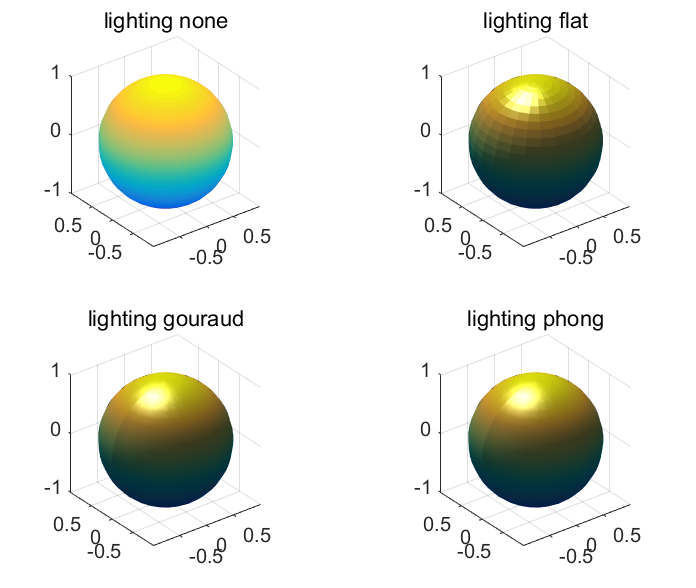lightangle函数：
lightangle(az,el)：az与el表示灯光的方位角和仰角light_handle = lightangle(az,el)：返回球形坐标光源的句柄值
sphere(25);
axis vis3d
h = light;
for az = -50:10:50
lightangle(h,az,30)
pause(.2)
end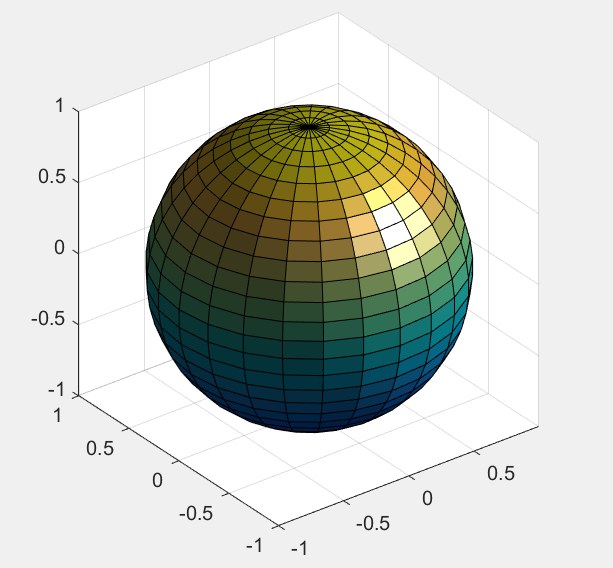5.等值线
等值线图又叫等高线图。默认情况下，MATLAB就是画出了相应于一系列相等的空间Z值得等值线。matlab提供了contour和contour3函数绘制二维和三维的等高线。下面的格式contour换成contour3就是三维的等值线。
contour(z)：直接绘制矩阵z的等高线contour(x,y,z)：用x和y指定等高线的x,y坐标contour(z,n)或contour(x,y,z,n)：用标量n指定绘制等高线的线条数，即从最低位置到最高位置所用的线条总数contour(z,v)或contour(x,y,z,v)：向量v中的元素指定绘制等高线的位置，该向量的长度对应绘制的线条数[c,h] = contour(...)：返回等高线矩阵c和列向量h，h是线条对象或补片对象的句柄。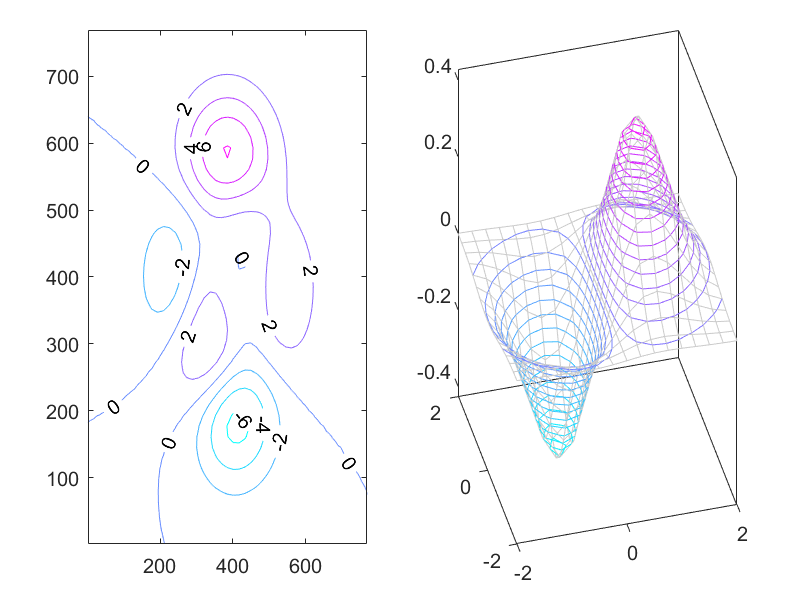6.三维特殊图形
MATLAB中，也提供了相应的函数用于实现特殊数的三维绘图。
t = 0:pi/10:2*pi;
[X1,Y1,Z1] = cylinder(2 + cos(t));
subplot(2,3,1);surf(X1,Y1,Z1)
axis square;title('三维柱面图');
subplot(2,3,2);sphere
axis equal;title('三维球体');
x1 = [1 3 0.5 2.5 2];
explode = [0 1 0 0 0];
subplot(2,3,3);pie3(x1,explode)
title('三维饼图');axis equal;
X2 = [0 1 1 2;1 1 2 2;0 0 1 1];
Y2 = [1 1 1 1;1 0 1 0;0 0 0 0];
Z2 = [1 1 1 1;1 0 1 0;0 0 0 0];
C = [0.5000 1.0000 1.0000 0.5000;
1.0000 0.5000 0.5000 0.1667;
0.3330 0.3330 0.5000 0.5000];
subplot(2,3,4);fill3(X2,Y2,Z2,C);
colormap hsv
title('三维填充图');axis equal;
[x2,y2] = meshgrid(-3:.5:3,-3:.1:3);
z2 = peaks(x2,y2);
subplot(2,3,5);ribbon(y2,z2)
colormap hsv
title('三维彩带图');axis equal;
[X3,Y3] = meshgrid(-2:0.25:2,-1:0.2:1);
Z3 = X3 .* exp(-X3.^2 - Y3.^2);
[U,V,W] = surfnorm(X3,Y3,Z3);
subplot(2,3,6);quiver3(X3,Y3,Z3,U,V,W,0.5);
hold on
surf(X3,Y3,Z3);
colormap hsv
view(-35,45);
title('三维向量场图');axis equal;
set(gcf,'Color','w');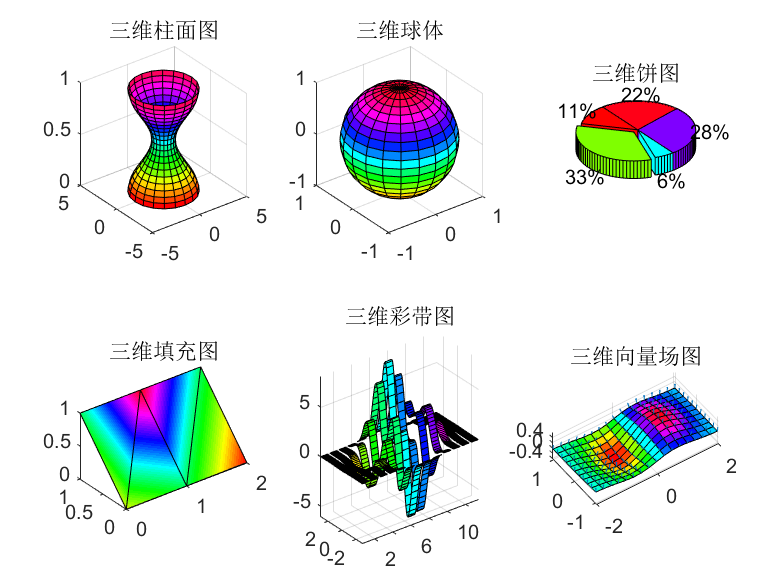7.视角设置
从不同的角度观察物体，所看到的物体形状是不一样的。同样，从不同视点绘制的三维图形其形状也是不一样的。
视点的位置可由方位角和仰角表示。方位角又称旋转角，它是视点与原点连线在xy平面上的投影与y轴负方向形成的角度，正值表示逆时针，负值表示顺时针。仰角又称为视角，它是视点与原点连线与xy平面的夹角，正值表示视点在xy平面上方。负值表示视点在xy平面下方。
matlab中提供了view和rotate函数用于设置观察图的视角。view函数用于调整图形的视角效果。
view(az,el)或view([az,el])：az带表方位角，el代表视角view([x,y,z])：在直角坐标中设置视角的坐标为（x,y,z）view(2)或view(3)：分别使用matlab中默认的二维视角设置(az=0,el=90)和三维视角设置(az=-38.5,el=30)view(ax,...)：使用ax轴代替当前轴显示图的视角[az,el] = view：返回图形的视角与俯视角值T = view：返回一个4x4阶的当前变换矩阵
x = -5:0.5:5;
[x,y] = meshgrid(x);
z = x.^2-y.^2-2;
subplot(2,2,1);surf(x,y,z);
view(-38.5,30);
title('方位角为-38.5，俯视角为30');
subplot(2,2,2);surf(x,y,z);
view(-38.5+90,30);
title('方位角为-38.5+90，俯视角为30');
subplot(2,2,3);surf(x,y,z);
view(-38.5,60);
title('方位角-38.5,俯视角为60');
subplot(2,2,4);surf(x,y,z);
view(180,0);
title('方位角为180，俯视角为0');
set(gcf,'color','w'); % 设置图形的背景颜色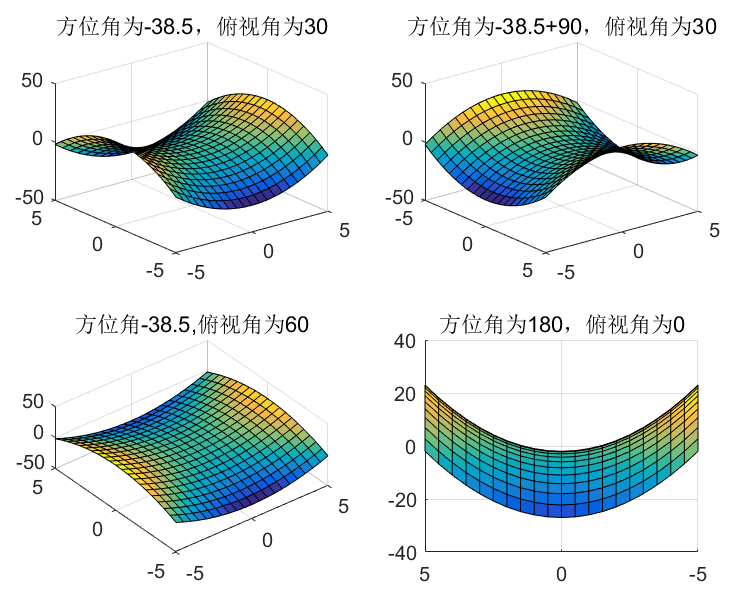rotate函数用于旋转三维图形，函数的格式为
rotate(h,direction,alpha)：将图形的句柄值h的对象绕方向旋转一个角度，h表示是被旋转的对；direction有两种设置方法，球坐标设置法，将其设置为[theta,phi]，其单位为‘度’；直角坐标法，也就是[x,y,z]；参数alpha是绕方向按照右手法则旋转的角度。rotate(...,origin)：参数origin为方向轴的“支点”坐标，系统默认为坐标原点。
sp11 = subplot(2,2,1);
hll = surf(sp11,peaks(20));
title('无旋转')
sp12 = subplot(2,2,2);
h12 = surf(sp12,peaks(20));
title('绕x轴旋转')
zdir = [1 0 0];
rotate(h12,zdir,25)
sp21 = subplot(2,2,3);
h21 = surf(sp21,peaks(20));
title('绕Y轴旋转')
zdir = [0 1 0];
rotate(h21,zdir,25)
sp22 = subplot(2,2,4);
h22 = surf(sp22,peaks(20));
title('绕X-Y轴旋转')
zidr = [1 1 0];
rotate(h22,zdir,25)
set(gcf,'color','w');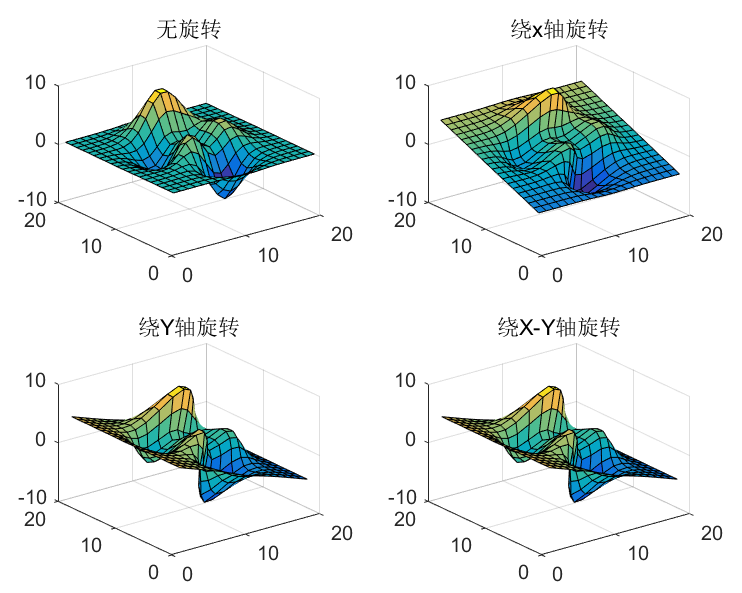二、四维绘图
在MATLAB中，提供了meshgrid、slice、contourslice函数，可充分体现四维图形的效果。
1.slice函数
matlab提供了中表现四维空间的方式，即使用色彩，这种方式需要用户调用slice函数来显示“切片”图。
slice(V,sx,sy,sz)：绘制立体V在x轴，y轴，z轴方向上与sx，sy，sz向量所对应点的切片图。其中V为mxnxp的三维立体数组。slice(X,Y,Z,V,X1,Y1,Z1)：沿着由数组X1,Y1与Z1定义的曲面绘制穿过立体V的切片。slice(...,'method')：指定内插的方法，method以下方法之一slice(axes_handle,...)：在句柄值axes_handle的坐标值中绘制立体切片图h = slice(...)：返回组成立体切片图的surface图形对象句柄值向量h.
[x,y,z] = meshgrid(-2:.2:2,-2:.25:2,-2:.16:2);
v = x.*exp(-x.^2-y.^2-z.^2);
xslice = [-1.2,.8,2];
yslice = 2;
zslice = [-2,0];
[xsp,ysp,zsp] = sphere;
slice(x,y,z,v,[-2,2],2,-2)
for i = -3:.2:3
hsp = surface(xsp + i,ysp,zsp);
rotate(hsp,[1 0 0],90)
xd = get(hsp,'XData');
yd = get(hsp,'YData');
zd = get(hsp,'ZData');
delete(hsp)
hold on
hslicer = slice(x,y,z,v,xd,yd,zd);
axis tight
xlim([-3,3])
view(-10,35)
drawnow
delete(hslicer)
hold off
end
2.contourslice函数
用于实现三元函数切面等高线的效果图。
contourslice(X,Y,Z,V,Sx,Sy,Sz)：X,Y,Z是维数为m x n x p的自变量“格点”数组；V是与X,Y,Z同维的函数值数组；Sx，Sy，Sz是决定切片位置的数值向量。假如取空阵，就表示不取切片。
[x y z v] = flow;
h = contourslice(x,y,z,v,[1:9],[],,linspace(-8,2,10));
axis([0,10,-3,3,-3,3]);daspect([1,1,1])
camva(24);
camproj perspective;
campos([-3,-15,5])
set(gcf,'Color',[.5,.5,.5],'Renderer','zbuffer')
set(gca,'Color','black','XColor','white',...
'YColor','white','Zcolor','white')
box on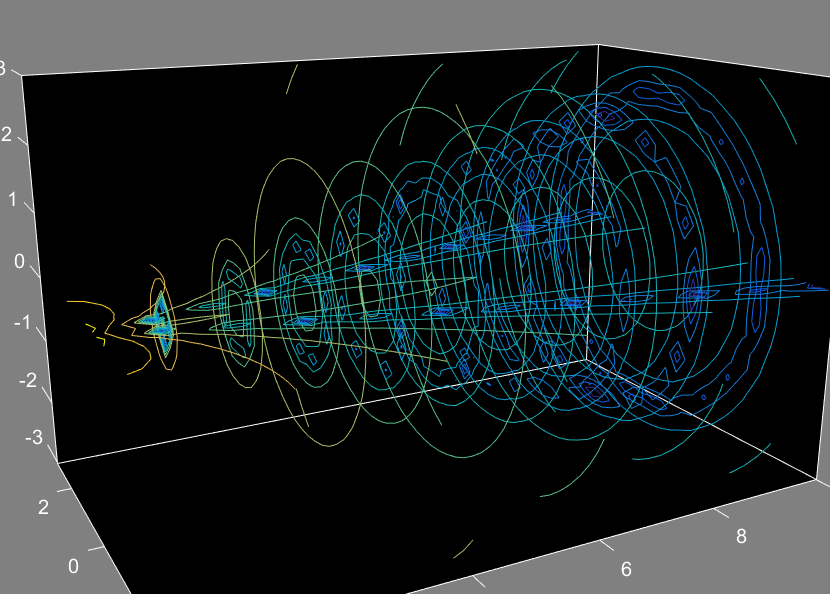展开全文基础 笔记
• 有许多不同的途径可以从这些数据提示游标中获得更多精确度：1)...我们假设你在默认变​​量cursor_info中导出它，然后得到一包含3字段的结构：cursor_info =Target: 492.0040Position: [7.3593e+05 10.6353]Dat...

有许多不同的途径可以从这些数据提示游标中获得更多精确度：
1)手动导出：
正如您在问题中提到的，右键单击数据提示，然后选择Export cursor data to workspace...。我们假设你在默认变​​量cursor_info中导出它，然后得到一个包含3个字段的结构：
cursor_info =
Target: 492.0040
Position: [7.3593e+05 10.6353]
DataIndex: 7
Target是光标对齐的line对象的句柄
Position是一个1x2向量，提供所选数据点的x和y坐标(如果定义了z坐标，它将具有第三个值)
DataIndex是用于绘制线的数组中所选数据点坐标的索引。所以你也可以要求：
>> x(7) %// or "x(cursor_info.DataIndex)" would be the same
ans =
7.3593e+05
>> y(7)
ans =
11.3200
现在默认情况下，控制台中值的显示限制为几位数，但变量中的数字具有更高的精度。 2种显示更高精度的方法：
第一种方法是在控制台中键入format long。之后，控制台中的所有数字输出都将是15位数字：
>> cursor_info.Position
ans =
1.0e+05 *
7.359289702215524 0.000106353183026
第二种方法是使用格式说明符sprintf或fprintf来强制所需的精度：
>> fprintf('x=%15.15d y=%g \n',cursor_info.Position)
x=7.359289702215524e+05 y=10.6353
2)以编程方式导出
除了手动导出cursor_info数据外，您还可以使用代码调用它：
dcm = datacursormode(gcf) ;
cursor_info = dcm.getCursorInfo ;
然后，您获得与手动导出相同的cursor_info变量。显示值的方式可以与上面相同。
3)直接显示(按照所需精度)。
您还可以完全自定义数据提示将显示的内容，不仅包括位置，还包括一些计算或转换的值。右键单击数据提示，然后选择Edit text update function。这将打开一个编辑器窗口，其中包含数据提示的当前代码，它基本上查询数据提示位置并创建要显示的文本单元格数组。根据需要修改此功能，然后将其保存在可以检索它的位置。
对于我正在使用的数据的示例，我以不同的精度显示x和y坐标，然后基于2个值进行计算，我还转换{{1}坐标到日期表示。
x
此示例将显示如下数据提示：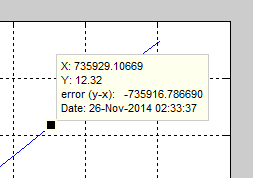之后，在任何后续的图或图中，您可以通过右键单击数据提示function output_txt = myModifiedDatatip(obj,event_obj)
pos = get(event_obj,'Position');
output_txt = {['X: ',num2str(pos(1),12)],... %// default code, only the output precision is changed
['Y: ',num2str(pos(2),8)]};
%// add all the informations you want to calculate and display directly here:
output_txt{end+1} = ['error (y-x): ',sprintf('%16f', pos(2)-pos(1))]; %// difference between x and x
output_txt{end+1} = ['Date: ', datestr(pos(1))]; %// display the date/time重新应用此数据提示格式，然后指向您之前保存的数据提示功能。

展开全文• 目录前言MATLAB插值函数绘图绘制立体曲线图绘制曲面图等高线在XY平面的投影 前言 调用一下MATLAB自带的seamount.mat数据文件 load seamount plot3(x,y,z,'.','markersize',12) xlabel('Longitude'), ylabel('...


目录
前言MATLAB插值函数三维绘图绘制立体曲线图绘制曲面图等高线在XY平面的投影

前言
调用一下MATLAB自带的seamount.mat数据文件
load seamount
plot3(x,y,z,'.','markersize',12)
xlabel('Longitude'), ylabel('Latitude'), zlabel('Depth in Feet')
grid on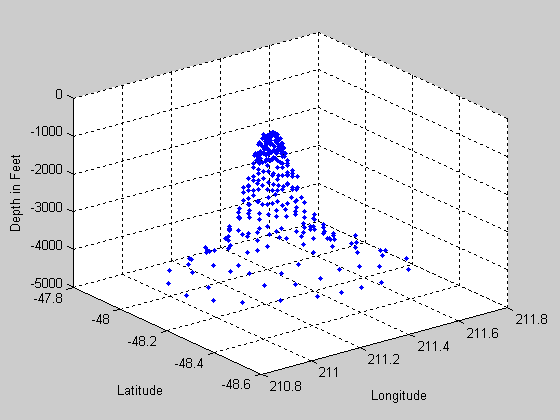采用函数griddata对三维数据进行插值，并通过变量xi和yi控制插值范围和数量
figure
[xi, yi] = meshgrid(210.8:0.01:211.8, -48.5:0.01:-47.9);
zi = griddata(x,y,z, xi,yi);
surf(xi,yi,zi);
xlabel('Longitude'), ylabel('Latitude'), zlabel('Depth in Feet')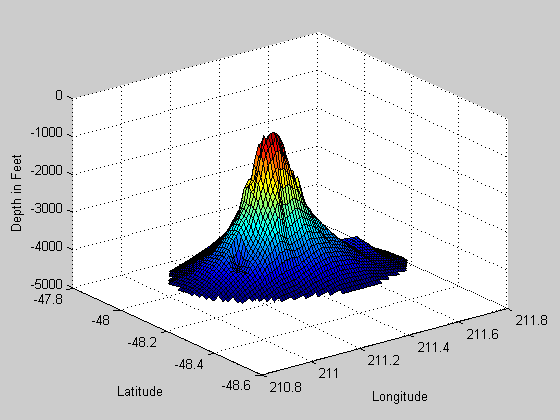MATLAB插值函数
这里引用一下论坛大佬对MATLAB插值函数的回复

2013b 中已经没有 griddata3 了，统一为 griddata，它能同时支持 2-D和3-D内插 （也就是已经包含了旧版本里的 griddata3 的功能）。请查看：http://www.mathworks.com/help/matlab/ref/griddata.html

另外 n >= 2 维的内插有一个新函数叫 griddatan，前面的 griddata 是 griddatan 取 n = 2 和 3 的两种特殊情形。griddatan 请参考：http://www.mathworks.com/help/matlab/ref/griddatan.html

还有一个TriScatteredInterp，同时支持 2-D 和 3-D 内插， 其效率比 griddata 更高，而且使用也更方便，因为它是返回的是一个内插式 （interpolant ） F， 类似函数句柄的东西 （但并非严格意义上的函数句柄），利用 F，可以直接计算网格点上的函数值。关于 TriScatteredInterp 的用法，请参考：http://www.mathworks.com/help/matlab/ref/triscatteredinterpclass.html?searchHighlight=TriScatteredInterp 不过，尽管 TriScatteredInterp 已经比 griddata 更有优势了，但是它在将来某个新版本中会被remove掉（参考至2013b的help文档：Note: TriScatteredInterp will be removed in a futurerelease. Use scatteredInterpolant instead.），取而代之的会是下面两个更强大的函数: scatteredInterpolant 和 griddedInterpolant

2012 里新增两个很有用的内插函数，scatteredInterpolant 和 griddedInterpolant，其中，scatteredInterpolant 支持 2-D 和 3-D 内插，就像 griddata/TriScatteredInterp 一样，但是功能更强大，它也是返回一个内插式 F（interpolant ），然后根据 F 可以求出内插点的函数值。请查看 http://www.mathworks.com/help/matlab/ref/scatteredinterpolantclass.html

griddedInterpolant 支持 n-D 内插，n = 1，2，3，4，5，。。。，这个类似于 griddatan，但功能更强大，它也是返回一个内插式 F（interpolant ），可以直接用F求内插点的函数值。请查看：http://www.mathworks.com/help/matlab/ref/griddedinterpolantclass.html

除了以上函数，再加上 interp1，interp2，interp3等，基本包含matlab里全部主流内插函数。
三维绘图
常用三维绘图指令如下（下图转自知乎）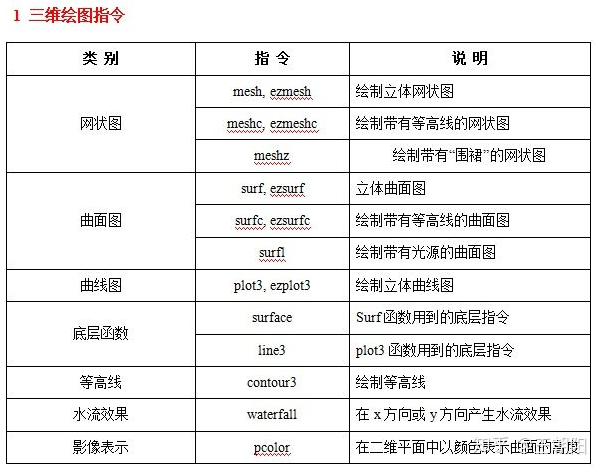指令多种多样，重点在于理解指令使用的内涵，一般常用的有画网状图的mesh，曲面图的surf和立体曲线图plot3。
绘制立体曲线图不需要建立两个单调递增的网格矢量，如[X,Y] = meshgrid(1:3,10:14)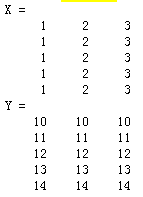绘制立体曲线图
t = 0:pi/50:10*pi;
plot3(sin(t),cos(t),t)
xlabel('sin(t)')
ylabel('cos(t)')
zlabel('t')
grid on
axis square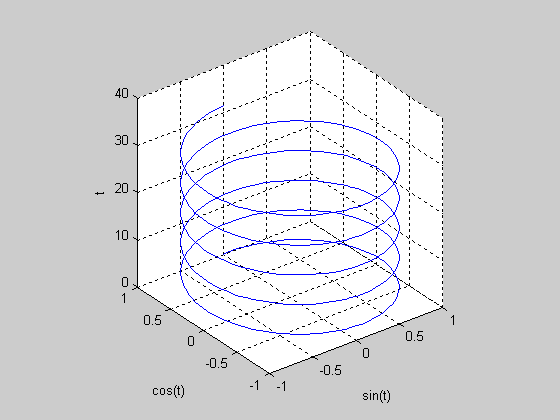绘制曲面图
k = 5;
n = 2^k-1;
[x,y,z] = sphere(n);%产生球，将坐标输入到变量
surf(x,y,z,c);
colormap([1  1  0; 0  1  1])
axis equal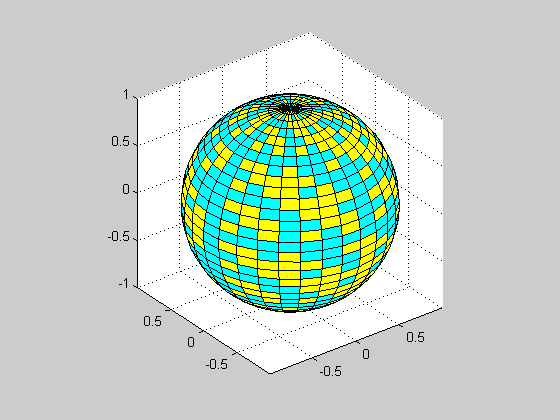等高线在XY平面的投影
加载MATLAB的数据，利用contour函数完成等高线图绘制。
load penny;
figure;
contour(flipud(P));
axis square;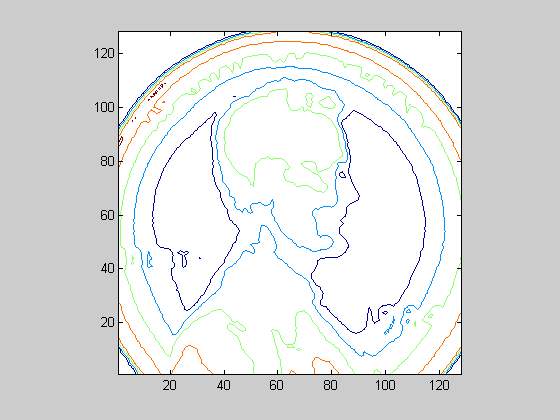展开全文• 每一量可能被当作数组，也可能被当作矩阵，这要依所采用的运算法则或运算函数 来定。在 MATLAB 中，数组与矩阵的运算法则和运算函数是有区别的。但不论是 MATLAB 的数组还是 MATLAB 的矩阵，都已经改变了...
• m文件中每callback都可以视为一独立执行的接口，也就说每callback之间无法做沟通操作，因此变量传递会有问题，有以下几种方式可以解决不同的回调函数之间的数据传递问题： 1.使用全局变量 当前有一组运算...gui
• 一、绘制维图像、 1、绘图步骤、 2、代码示例
• matlab开发-多变量计算工具箱Ver19。这是一个处理三个变量的标量和向量函数的工具箱。容易绘图等…
• MATLAB中，变量名是以字母开头，后接字母、数字或下画线的字符序列，最多63字符。 错误示例 正确示例 注意 变量名区分字母的大小写。（大写的A和小写的a是两不同的变量MATLAB提供的标准函
• 基础语法查阅w3cschool的MATLAB教程，学习基本语法MATLAB 教程_w3cschool​m.w3cschool.cntip:如果语句后面不加分号，matlab会以交互式来执行程序，所以每执行一步，都会输出，而加了分号，matlab会执行这语句，...
• ( )(A)_num (B)num_ (C)num- (D)-num 2、 在MATLAB中，要给出一复数z的模，应该使用( )函数。(A)mod(z) (B)abs(z) (C)double(z) (D)angle(z) 3、 下面属于MATLAB的预定义特殊变量的是？( )(A)eps (B)no...
• matlab绘图维图形维曲线plot3函数 维曲线 plot3函数 plot（x,y,z） x,y,z通常为长度相等的向量mesh surf plot3 程序人生
• 假设数据是a,b,c三个列如果使用plot3(a,b,c)，将得到 使用 [X,Y] = meshgrid(b,c); [~,Z] = meshgrid(a); figure;surf(Z,X,Y); 得到 使用插值的话 [X,Y]=meshgrid(linspace(min(b),max(b),100),linspace...曲面插值
• ## 【Matlab】MATLAB绘图

万次阅读 多人点赞 2018-06-29 17:18:39
专题四 MATLAB绘图 绘图的目的是使数据可视化。 一 二维曲线 1. 函数plot() 在MATLAB中，函数plot()是最基本的绘图函数，利用它可以绘制出不同的二维曲线。函数plot()的基本用法： plot(x, y) % 其中，x和y...
• m文件中每callback都可以视为一独立执行的接口，也就说每callback之间无法做沟通操作，因此变量传递会有问题，有以下几种方式可以解决不同的回调函数之间的数据传递问题： 1.使用全局变量 当前有一组...gui
• MATLAB三绘图(三)绘制等值线图 1、使用contour函数绘制等值图，示例： %% 显示不同颜色的梯度图 clear; clc; close all; x = -3.5:0.2:3.5; y = -3.5:0.2:3.5; [X,Y] = meshgrid(x,y); % 获取网格 Z = X.*exp(-X....
• 三维绘图即包含三个变量，通常分为两种： 1）一个独立变量t，两外两个变量x,y随t变化。比如曲线的参数方程描述 2）两个独立变量x,y,第三个变量z随x,y变化。比如z=x^2+y^2 三维绘图函数主要有plot3、mesh、surf、...
• 1、特殊变量与常数 ans 计算结果的变量名 computer 确定运行的计算机 ...nargin 输入参数数 nargout 输出参数的数目 pi 圆周率 nargoutchk 有效的输出参数数目 realmax 最大正浮点数 realm
• 成果图如上图所示，黑色的线，红色的线和绿色的线分别代表数据集中的三个标签类别，横轴代表样本总数90个（图中没有截全，只能看到40左右），纵轴代表每个样本的长度256个点。 主要实现函数（plot3） 步骤分为： 1....plot3 二维数组
• 强大的绘图功能是Matlab的特点之一，Matlab提供了一系列的绘图函数，用户不需要过多的考虑绘图的细节，只需要给出一些基本参数就能得到所需图形，这类函数称为高层绘图函数。此外，Matlab还提供了直接对图形句柄...python webgl 数学建模 opengl
• 相关问题安全标志分为四类，它们分别是 __ 。: 标志 标志 警告 标志 标志 提示 标志在AutoCAD中用尺寸标注命令所形成的尺寸文本,尺寸线和尺寸界线类似于块,可以用EXPLODE命令来分解: AutoCAD 中 尺寸 标注 命令 尺寸...
• ## Matlab绘图

千次阅读 2018-05-27 10:36:24
强大的绘图功能是Matlab的特点之一，Matlab提供了一系列的绘图函数，用户不需要过多的考虑绘图的细节，只需要给出一些基本参数就能得到所需图形，这类函数称为高层绘图函数。此外，Matlab还提供了直接对图形句柄进行...
• 如何在matlab上生成这两个变量取值范围的临界线图形(二维图形，横纵坐标分别是x,y)？根据题主给的函数z=x+y≥10，其中x≥3，y≥1为例，说明求解过程：第一步，分别对x、y进行赋值，且满足已知条件，即x=linspace(3,...
• 赋值语句二、预定义变量三变量的管理1.内存变量的修改与删除2.who&whos命令3.内存变量文件 一、变量与赋值语句 1.变量 变量：内存单元的一抽象， 关于变量名的说明： ——变量名是以字母开头，后接字母，...线性代数 矩阵
• 欢迎关注工科男的Maltab学习日志，采用...——工科男MATLAB符号计算1 符号计算基础1.1符号运算1.2 符号对象1.3 符号表达式中变量的确定2 符号导数及其应用2.1函数的极限2.2 符号函数求导及其应用3 符号积分4 级数...
• MATLAB 绘图 线型 颜色 标记 粗细 Harry Potter Gryffindor 90 Hermione Granger Gryffindor 100 Draco Malfoy Slytherin 90 MATLAB 函数 函数 调用格式......

# matlab三个变量绘图matlab 订阅